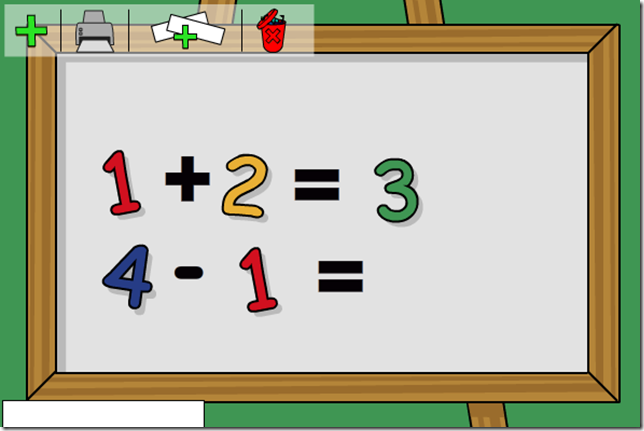Select PageThe Langeroo Numbers Topic has been updated and improved! To the Scene Maker Tool we have added a zero and the mathematical signs for add, subtract, multiply, divide and equals. These new features will enable teachers to present a variety of mathematical concepts in a fun, easy to use way.

Discussing and talking about mathematics and mathematical concepts is a key and vital part of children’s learning and understanding of maths. It is also important that teachers use a variety of media to represent different mathematical information, and for children have opportunities to talk about and discuss maths.

Below are some examples of how the Langeroo Numbers Scene Maker Tool may be used:

• Sequence numbers
• Number names
• Recite numbers in order from and back to zero
• Odd and even
• Place value
• Approximation
• Number bonds
• Mathematical language
• Recognise the signs +, -, x, ÷ and = and understand their meaning
• Calculate simple +, -, x and ÷

We love to hear how our users are using Langeroo in cross-curriculum situations.  Please, drop us a line and let us know how you’re getting on!